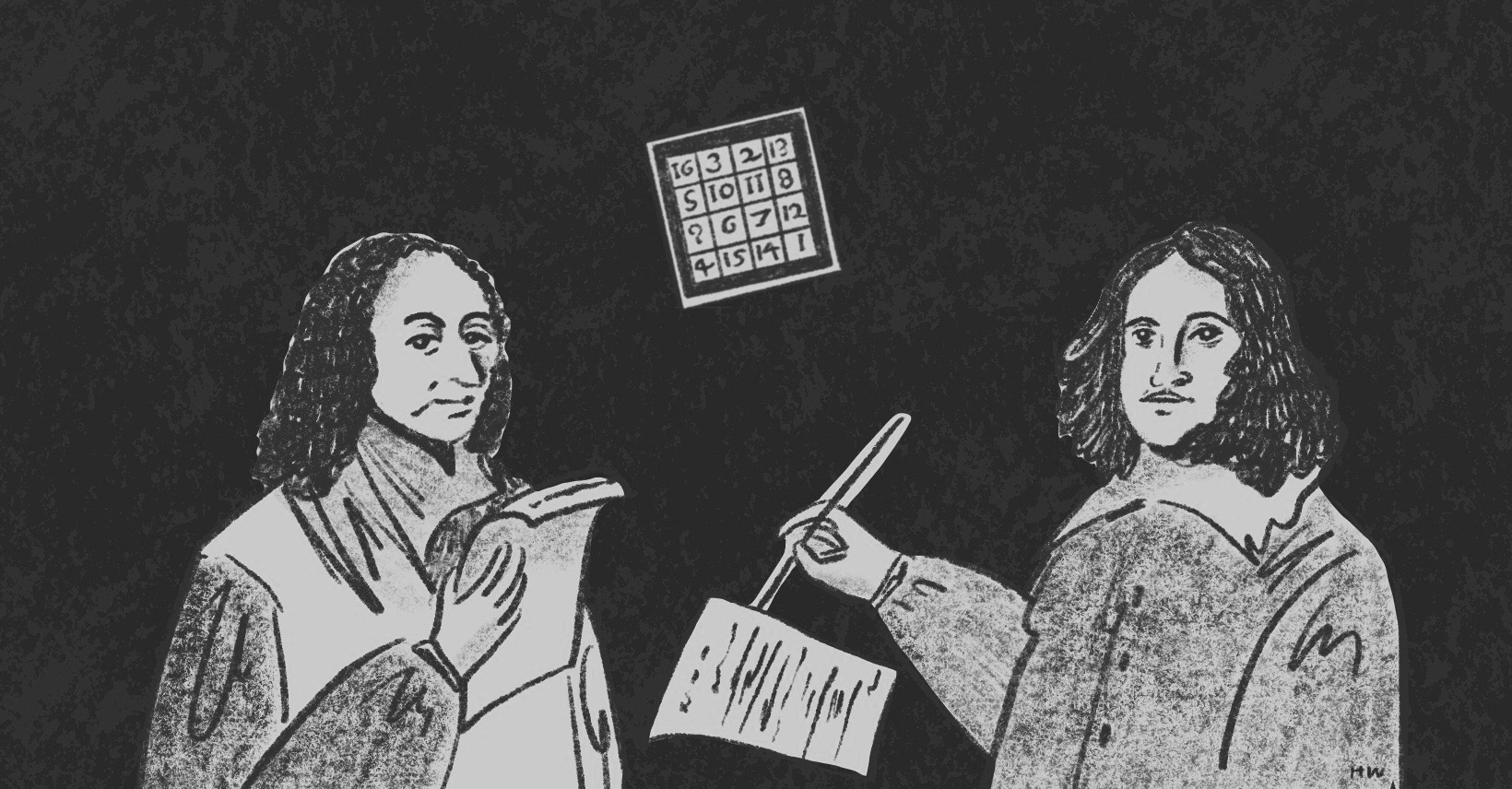×資料管理 統計分析 相關資源 巨人肩膀 語法索引 關於作者

## 簡介

• aov() -變異數分析
• shapiro.test() -Shapiro常態分配檢定
• mshapiro.test() -多變量Shapiro常態分配檢定
• bartlett.test() -Bartlett變異數同質性檢定
• leveneTest() -Levene變異數同質性檢定
• fligner.test() -Fligner-Killeen變異數同質性檢定
• boxM() -Box's M矩陣共變數同質性檢定
• scheffe.test() -Scheffe事後檢定
• TukeyHSD() -Tukey事後檢定
• manova() -多變量變異數分析

• 常態性：樣本必須符合常態分配，也就是分配至少要長得差不多，奇形怪狀的分配是沒辦法比較的。
• 獨立性：樣本要有獨立性，也就是抽樣一定要隨機。
• 同質性：各組變異數必須相等或接近，也就是兩組常態分配曲線的高矮胖瘦要差不多，差太多代表長得不一樣，不一樣的東西怎麼能比較呢。

$$SS_{B}=\displaystyle\sum_{i=1}^{k}n_{i}(\overline{Y}_{i}-\overline{\overline{Y}})^2$$ (公式2)
k-1 $$MS_{B}=\frac{SS_{B}}{k-1}$$ $$\frac{MS_{B}}{MS_{W}}$$

$$SS_{W}=\displaystyle\sum_{i=1}^{k}(n_{i}-1)S_{i}^{2}$$ (公式2)
n-k $$MS_{W}=\frac{SS_{W}}{n-k}$$

## 單因子變異數分析 One-Way ANOVA

 > rate<-read.csv("c:/Users/USER/downloads/tv_rate.csv", header=TRUE, sep=",") > str(rate) #觀看資料結構 'data.frame': 30 obs. of 8 variables: $Channel : chr "中視" "台視" "東森新" "TVBN" ...$ Program : chr "中視新聞全球" "台視晚間新聞" "1800東森晚間" "九點熱話題" ... $Type : chr "news" "news" "news" "news" ...$ Schedule: chr "19:00" "19:00" "17:45" "21:00" ... $Time : chr "prime" "prime" "normal" "normal" ...$ Rate : num 2.45 2.18 1.86 1.58 1.56 1.53 1.43 1.23 1.17 1.02 ... $CPM : int 67 75 93 118 119 97 108 121 133 241 ...$ AdvCost : int 33000 33000 35000 37500 37500 30000 31250 30000 31250 49500 ... > tapply(rate$Rate, rate$Type, mean) #不同節目類型的平均收視率 drama news show & cartoon 0.6357143 1.2121429 0.4711111 > tapply(rate_sample$Rate, rate_sample$Type, var) #不同節目類型的收視率變異數 drama news show & cartoon 0.17372857 0.45691044 0.07116111 

### 分析結果

 > oneway<-aov(Rate~Type, data=rate) #將ANOVA結果指定給oneway > summary(oneway) #輸出分析結果 Df Sum Sq Mean Sq F value Pr(>F) Type 2 3.449 1.7243 6.165 0.00623 ** Residuals 27 7.551 0.2797 --- Signif. codes: 0 ‘***’ 0.001 ‘**’ 0.01 ‘*’ 0.05 ‘.’ 0.1 ‘ ’ 1 

### 同質性檢定

 > library(car) #載入car套件 > leveneTest(Rate~Time, data=rate) #Levene變異數同質性檢定 Levene's Test for Homogeneity of Variance (center = median) Df F value Pr(>F) group 1 0.7414 0.3965 28 Warning message: In leveneTest.default(y = y, group = group, ...) : group coerced to factor. > fligner.test(Rate~Time, data=rate) #Fligner-Killeen變異數同質性檢定 Fligner-Killeen test of homogeneity of variances data: Rate by Time Fligner-Killeen:med chi-squared = 1.4877, df = 1, p-value = 0.2226 

Levene或Fligner-Killeen檢定的p值>.05，代表變異數同質。接下來進行雙因子變異數分析。

### 分析結果

 > twoway<-aov(Rate~Type*Time, data=rate) #雙因子變異數分析含Type*Time交互作用項 > summary(twoway) Df Sum Sq Mean Sq F value Pr(>F) Type 2 3.449 1.7243 5.878 0.00836 ** Time 1 0.247 0.2466 0.841 0.36829 Type:Time 2 0.265 0.1324 0.451 0.64203 Residuals 24 7.040 0.2933 --- Signif. codes: 0 ‘***’ 0.001 ‘**’ 0.01 ‘*’ 0.05 ‘.’ 0.1 ‘ ’ 1 

### Box's M 同質性檢定

 > library(heplots) #載入heplots套件 > boxM(y~Type, data=rate) Box's M-test for Homogeneity of Covariance Matrices data: Y Chi-Sq (approx.) = 16.017, df = 6, p-value = 0.01366 

Box's M檢定共變數矩陣並不符合同質性假設，但為說明方便，我們還是繼續用本例進行MANOVA。

 > manova<-manova(y~Type, data=rate) > summary(model) Df Pillai approx F num Df den Df Pr(>F) Type 2 0.43134 3.7121 4 54 0.009671 ** Residuals 27 --- Signif. codes: 0 ‘***’ 0.001 ‘**’ 0.01 ‘*’ 0.05 ‘.’ 0.1 ‘ ’ 1 

### 分析結果

R預設的MANOVA統計檢定是Pillai's trace，其實常用的方法有Pillai, Wilks, Hotelling-Lawley, Roy。可以透過summary()選擇統計方法：

 > summary(manova(y~Type), test="Wilks") > summary(manova(y~Type), test="Hotelling-Lawley") > summary(manova(y~Type), test="Roy") 

—Pillai's trace: $$\displaystyle\sum_{k=1}^{k} \frac{\lambda_{k}}{1+\lambda_{k}}$$，數字越大表示對模型貢獻度越大。
—Wilk' Lambda: $$\displaystyle\prod_{k=1}^{k} \frac{1+\lambda_{k}}{1}$$，0-1的統計量，與Pillai's trace相反，數字越小表示對模型貢獻度越大。
—Hotelling's trace: $$\displaystyle\sum_{k=1}^{k}{\lambda_{k}}$$，矩陣特徵值的總和，數字越大表示對模型貢獻度越大，數值通常會大於Pillai's trace。
—Roy's largest root: $$\lambda_{max}$$，矩陣中最大的特徵值，數字越大表示對模型貢獻度越大，數值通常會小於Hotelling's trace。

### 追蹤檢驗 Follow-up Analysis

MANOVA結果，節目型態達到統計顯著。接下來我們要問的是，節目型態到底是和哪一個應變數達到顯著？也就是所謂的追蹤檢驗(follow-up analysis)，可以利用summary.aov( )獲得檢定統計量。這裡只顯示Pillai檢定的結果，節目型態與收視率達到顯著，但廣告費則不顯著。

 > summary.aov(model, test="Pillai") Response 1 : Df Sum Sq Mean Sq F value Pr(>F) Type 2 3.4487 1.72433 6.1652 0.006231 ** Residuals 27 7.5515 0.27969 --- Signif. codes: 0 ‘***’ 0.001 ‘**’ 0.01 ‘*’ 0.05 ‘.’ 0.1 ‘ ’ 1 Response 2 : Df Sum Sq Mean Sq F value Pr(>F) Type 2 251295238 125647619 0.9776 0.3891 Residuals 27 3470321429 128530423 --- Signif. codes: 0 ‘***’ 0.001 ‘**’ 0.01 ‘*’ 0.05 ‘.’ 0.1 ‘ ’ 1 > summary.aov(model, test="Wilks") > summary.aov(model, test="Hotelling-Lawley") > summary.aov(model, test="Roy")